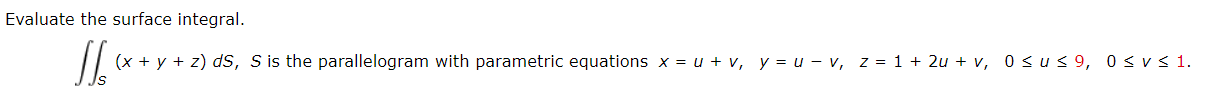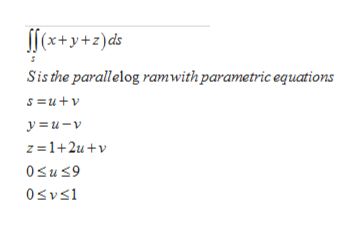# Evaluate the surface integral.(x + y + z) dS, S is the parallelogram with parametric equations x = u + v, y = u – v, z = 1 + 2u + v, 0sus 9, 0sv< 1.

Question
16 viewshelp_outlineImage TranscriptioncloseEvaluate the surface integral. (x + y + z) dS, S is the parallelogram with parametric equations x = u + v, y = u – v, z = 1 + 2u + v, 0sus 9, 0sv< 1. fullscreen
check_circle

Step 1

Evaluate the surfa...help_outlineImage Transcriptionclose[(x+y+z)ds Sis the parallelog ramwith parametric equations s =u+v y = u – v z =1+2u +v Osus9 Osvs1 fullscreen

### Want to see the full answer?

See Solution

#### Want to see this answer and more?

Solutions are written by subject experts who are available 24/7. Questions are typically answered within 1 hour.*

See Solution
*Response times may vary by subject and question.
Tagged in

### Calculus